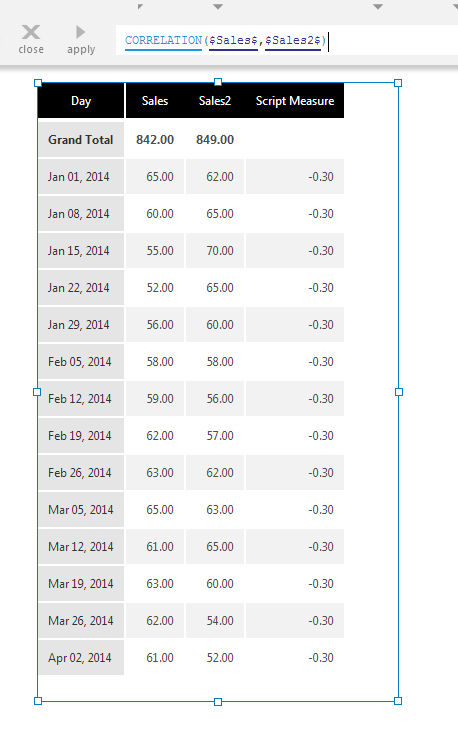# Correlation

Contents[Hide]

The CORRELATION function calculates the correlation, or the strength of the relationship between two input data series.

The resulting correlation value ranges between -1 and 1, where a value close to -1 indicates a negative correlation, a value close to 1 indicates a positive correlation, and a value close to zero indicates no correlation. A negative correlation means that when the first input is large, the second input is small (and vice-versa) whereas a positive correlation means the both input values become larger and smaller at the same time.Correlation shown on the Script Measure column

## 1. Syntax

```CORRELATION(d0,d1,Alignments...)
```

## 2. Input

The Correlation function requires the following input series:

• d0: First Input data values - The first set of data values for which the Correlation is calculated.
• d1: Second Input data values - The second set of data values for which the Correlation is calculated.

## 3. Parameters

The Correlation function requires the following parameters:

• Alignments... (One or More, Optional) – Hierarchy placeholders to be used as the alignment axis.

## 4. Output

The Correlation function generates the following outputs:

• Correlation - A single value representing the correlation between the two sets of input values.